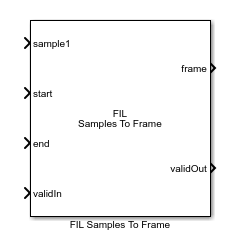# FIL Samples To Frame

Convert sample stream from FPGA-in-the-loop to frame-based data

• Library:
• Wireless HDL Toolbox / I/O Interfaces

•## Description

The FIL Samples To Frame block performs the same sample-to-frame conversion as the Samples To Frame block. It accepts input data as vectors of the entire frame of samples. The block expects control signal input vectors of the same width as the sample data. This optimization speeds up the communication link between the FPGA board and your Simulink® simulation when using FPGA-in-the-loop. To run FPGA-in-the-loop, you must have an HDL Verifier™ license.

When you generate a programming file for a FIL target in Simulink, the tool creates a model to compare the FIL simulation with your Simulink design. For Wireless HDL Toolbox™ designs, the FIL block in that model replicates the sample-streaming interface to send one sample at a time to the FPGA. You can modify the autogenerated model to use the FIL Frame To Samples and FIL Samples To Frame blocks to improve communication bandwidth with the FPGA board by sending one frame at a time. For how to modify the autogenerated model, see FPGA-in-the-Loop.

## Ports

### Input

expand all

Stream of samples, specified as a vector representing an entire frame. The stream can include idle cycles between samples and between frames. Idle samples are discarded.

If you set Number of input samples greater than one, the block shows one port for each input value. In this case, a single sample is represented by N values, such as turbo-encoded samples represented by one systematic value and two parity values. The input data is one vector for each port. The control signals apply to all sampleN ports.

Data Types: `single` | `double` | `int8` | `int16` | `int32` | `uint8` | `uint16` | `uint32` | `Boolean` | `fixed point`

Start of frame, specified as a `Boolean` vector containing one value for each sample in the frame. This signal is 1 (true) for one timestep corresponding to the first valid sample of the frame.

Data Types: `Boolean`

End of frame, specified as a `Boolean` vector containing one value for each sample in the frame. This signal is 1 (true) for one timestep corresponding to the last valid sample of the frame.

Data Types: `Boolean`

Validity of samples, specified as a `Boolean` vector containing one value for each sample in the frame. This signal is 1 (true) on timesteps that correspond to valid samples.

Data Types: `Boolean`

### Output

expand all

Frame of output samples, returned as a column vector. Each frame is the same size. If the input frame is smaller than Output size, the block pads the frame with zeroes. If the output frame is larger than the Output size, the block forms the frame by using the first Output size samples. You can optionally output the number of valid samples in each frame on the len port.

Validity of output frame, returned as a `Boolean` scalar. This port returns `1` (`true`) when the values on the frame and len (optional) ports, are valid.

Data Types: `Boolean`

Number of valid samples in output frame, returned as an integer. The input sample stream can have frames of different sizes. The block returns a constant size vector on the frame port, padded with zeroes when the input frame is smaller than Output size. The len port indicates how many valid samples are in the output frame. If the output frame is larger than the Output size, the block forms the frame by using the first Output size samples.

Data Types: `double`

## Parameters

expand all

Number of values representing each sample, specified as a positive integer scalar. The block has one sampleN port for each value. The control signals apply to all sampleN ports. For example, you can use this parameter for turbo-encoded samples. In the LTE standard, the turbo code rate is 1/3, so each sample is represented by one systematic value and two parity values: S_n, P1_n, and P2_n. In this case, set Number of input samples to `3`.

Maximum number of samples per frame, specified as an integer. The input sample stream can have frames of different sizes. The block returns a constant size vector, padded with zeroes if the frame is smaller than Output size. If the block receives a frame larger than Output size, it truncates the frame.

Order of output samples relative to input order, when more than one value represents each sample. For example, 1/3 turbo-encoded samples are represented by `[S_1 P1_1 P2_1]` and ```[S_2 P1_2 P2_2]```. The default output order is ```[S_1 P1_1 P2_1 S_2 P1_2 P2_2]```. To reorder the samples so that systematic and parity values are grouped together, select Compose output from interleaved input samples. The output order is then `[S_1 S_2 P1_1 P1_2 P2_1 P2_2]`.

Enable frame length output port. Select this option to return the number of valid samples in each output frame. The length is returned on the len port and is qualified by the valid signal. Use this option when the sample stream has variable size frames or when a downstream block requires the frame size as input, such as LTE Turbo Decoder.

## Version History

Introduced in R2017b